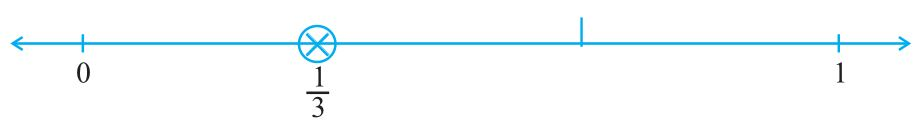# Chapter 7 Fractions Class 6 Notes Maths

Here you will find Chapter 7 Fractions Class 6 Notes Maths that will help the students to recall information with more precision and faster. You will understand the various factors through which one can improve their efficiency and eventually scores higher in the exam. You will also find NCERT Solutions for Class 6 Chapter 7 Maths which will help you in improving the marks in the examinations. Class 6 NCERT Revision Notes becomes a vital resource for all the students to self-study from NCERT textbooks carefully.Fractions

• Fraction is a number representing part of a whole. The whole may be a single object or a group of objects.

Fractions on the number line

• In order to show 1/3 on a number line. We divide the length between 0 and 1 into 3 equal parts and show one part as 1/3.Proper Fractions

• A proper fraction is a number representing part of a whole.

• In a proper fraction the denominator shows the number of parts into which the whole is divided and the numerator shows the number of parts which have been considered. Therefore, in a proper fraction the numerator is always less than the denominator.

Improper Fractions

• The fractions where the numerator is bigger than the denominator are called improper fractions.

Mixed Fractions

• A mixed fraction has a combination of a whole and a part.

• We can express an improper fraction as a mixed fraction by dividing the numerator by denominator to obtain the quotient and the remainder. Then the mixed fraction will be written as Quotient × Remainder/Divisor.

Equivalent Fractions

• Two or more fractions representing the same part of a whole.

• To find an equivalent fraction of a given fraction, you may multiply both the numerator and the denominator of the given fraction by the same number. For example,
Equivalent fractions of 1/3 are 2/6, 3/9.

• To find an equivalent fraction, we may divide both the numerator and the denominator by the same number. For example,
Equivalent fraction of 12/15 is 4/5.

Simplest Form of a Fraction

• A fraction is said to be in the simplest (or lowest) form if its numerator and denominator have no common factor except 1.

• The shortest way to find the equivalent fraction in the simplest form is to find the HCF of the numerator and denominator, and then divide both of them by the HCF.

Like Fractions

• Fractions with same denominator is called Like Fractions.

• Comparing Like Fractions: Between two like fractions with the same denominator, the fraction with the bigger numerator is greater. For example, 2/5 < 3/5 < 4/5.

• Addition and Subtraction of Like Fractions: You can do these operations just by adding or subtracting the numerators.

Unlike Fractions

• Fractions with different denominator is called Unlike Fractions.

• Addition and Subtraction of Unlike Fractions: You can do these operations by converting them into like fractions and adding or subtracting the numerators.

• Comparing Unlike Fractions: There are two ways with same numerator and different numerator.
→ Unlike Fractions with the same numerator: If the numerator is the same in two fractions, the fraction with the smaller denominator is greater of the two. For example, 2/3 < 2/5.
→ Unlike Fractions with the different numerator: We can compare them by converting into like fractions. Or we can cross multiply and check.

For example, Compare 4/5 and 5/6

4 × 6 /5 × 6 = 24/30 and 5× 5 /6 × 5 = 25/30
= 24/30 < 25/30.

Convert Like Fractions into Unlike Fractions

• First find the LCM (lowest common multiple) of the denominators of the given fractions.
• Then convert each fraction to its equivalent fraction with denominator equal to the LCM obtained in previous step.